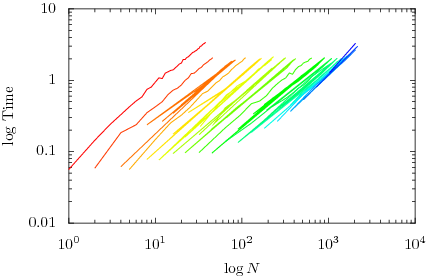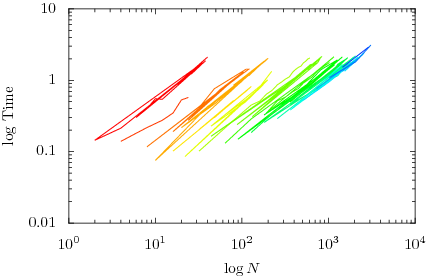# Benchmarking CouchDB (1)

I’ve written a small benchmark for couchdb to test it’s document creation performance. A script creates $$N$$ documents in total using bulk update to create $$B$$ at the same time with $$T$$ concurrent threads. The following graph show the time it takes to create an amount of documents against that amount of document for different values of $$B$$ with $$T=1$$.And for $$T=2$$ (two concurrent threads. Tested on a dual core machine)The values of B are 1, 2, 4, 5, 8, 11, 16, 22, 32, 45, 64, 90, 128, 181, 256, 362, 512, 724 and 1024

As you can see, a higher value of $$B$$ causes the graph to shift to the right which means more $$N$$ for the same time. Bulk update really does make a difference. Or non-bulk-update really sucks. Also adding threads does help a bit, but not as much as expected.

There are some more interesting graphs to plot ($$B$$ against $$\overline {N \over \Delta T}$$). More graphs tomorrow.

(For those interested, the raw data from which these graphs were plotted.)